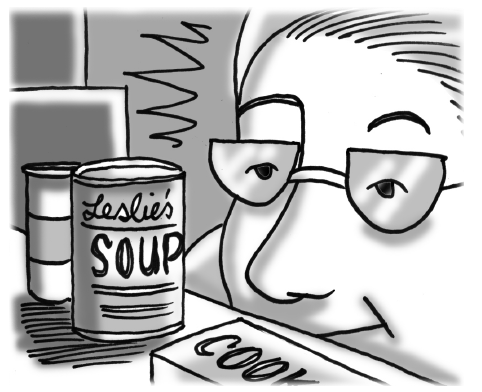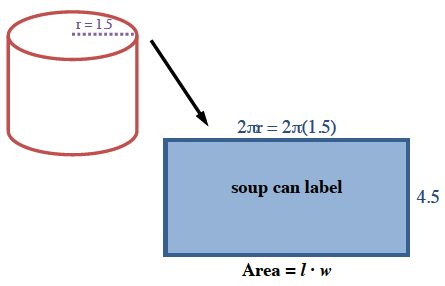### Home > GC > Chapter 11 > Lesson 11.1.3 > Problem11-42

11-42.While volunteering for a food sale, Aimee studied a cylindrical can of soup. She noticed that it had a diameter of $3$ inches and a height of $4.5$ inches.

1. Find the volume of the soup can.

$\text{Volume}=\pi r^2\cdot h$

$31.81$ cubic inches

2. If Aimee needs to fill a cylindrical pot that has a diameter of $14$ inches and a height of $10$ inches, how many cans of soup will she need?

$\text{Number of cans Aimee will need }= \frac{\text{volume of the pot}}{\text{volume of the soup can}}$

3. What is the area of the soup can label?